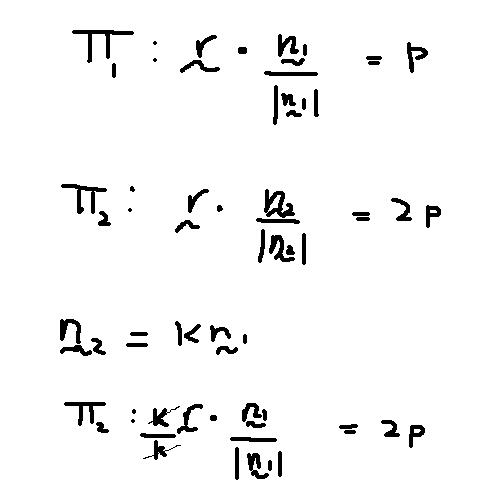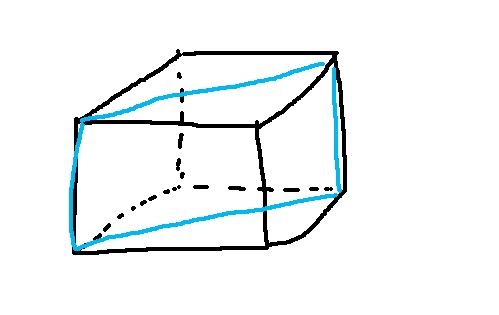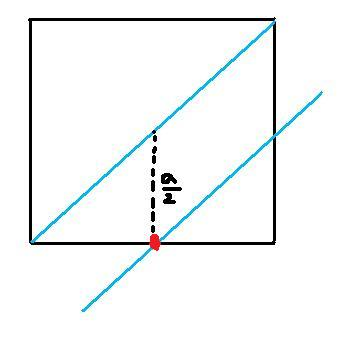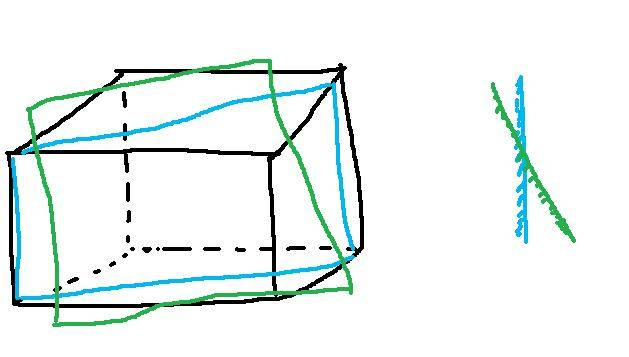# Perpendicular distance between planes

unscientific

## Homework Statement

The question is as attached in the picture.

## The Attempt at a Solution

I understand and got the answer to part (a) but from (b) onwards I am completely lost. Here are my assumptions:

1)Edges of 'cube' are still of length a

2)Successive planes are parallel

But, there is some things remain unknown:

1)Where is the origin at? The centre of the cube? or at the bottom leftmost point?

2)Why does the plane pass through (a/2, 0, a/2)?

3) Do we assume that the plane contains the origin? Then won't the equation be

r . n = 0?

#### Attachments

•cube1.jpg
27.8 KB · Views: 493
Last edited:

unscientific
would appreciate any help..

voko
1)Where is the origin at? The centre of the cube? or at the bottom leftmost point?

The description states, the center of the cube.

2)Why does the plane pass through (a/2, 0, a/2)?

There is an atom at (a/2, 0, 0) through which a plane has to pass; so it passes through (a/2, 0, z) for any z because it is parallel to the z axis. I am not sure why they did not specify the obvious point, though.

3) Do we assume that the plane contains the origin? Then won't the equation be

r . n = 0?

It is another plane that satisfies the equation, but not for a positive p. They actually use this plane implicitly when they claim that the p found is the distance between the planes. Can you see how?

unscientific
There is an atom at (a/2, 0, 0) through which a plane has to pass; so it passes through (a/2, 0, z) for any z because it is parallel to the z axis. I am not sure why they did not specify the obvious point, though.

Based on the face-centred structure, there is no atom at (a/2, 0, a/2) as that 'centre-face' atom only exists on alternate layers...

So what I would do is:

a. Taking the plane 1 to be the plane containing origin,

b. Find equation of plane 3 (that contains the atom at (a/2, 0, a) ).

c. Find distance between plane 3 and plane 1 then divide by 2.

This gives me a distance of a/sqrt(32), which is half of what the answer has..

(this is assuming the vertical height between layers is always a/2)

This whole question can be resolved if it said something like "All layers of the crystal are arranged in the same manner". Then it will no longer be alternate layers..

Last edited:
voko
This gives me a distance of a/sqrt(32), which is half of what the answer has..

I don't see how you get this. $$\frac 1 {\sqrt{2}} (1, 1, 0) (\frac a 2, 0, a) = \frac 1 {\sqrt{2}} (1 \cdot \frac a 2 + 1 \cdot 0 + 0 \cdot a) = \frac 1 {\sqrt{2}} \frac a 2 = \frac a {\sqrt{8}}$$

unscientific
I don't see how you get this. $$\frac 1 {\sqrt{2}} (1, 1, 0) (\frac a 2, 0, a) = \frac 1 {\sqrt{2}} (1 \cdot \frac a 2 + 1 \cdot 0 + 0 \cdot a) = \frac 1 {\sqrt{2}} \frac a 2 = \frac a {\sqrt{8}}$$

This is the perpendicular distance between the 1st and 3rd layer....So We must divide by 2

voko
Why is it 3rd? Where is the second?

unscientific
Why is it 3rd? Where is the second?

Because looking at the cubic structure, the atom that is in the centre of a face occurs only at every alternate layer..

UNLESS

every layer is the same..

voko
I do not think we are getting anywhere. The first plane with the given normal vector passes through the atom at (0, 0, a/2), and that plane passes through the origin. There is a plane that passes through (a/2, 0, 0), which also passes through (a/2, 0, a). You say this the third plane. Where is the second plane? What atom does it pass through?

unscientific
I do not think we are getting anywhere. The first plane with the given normal vector passes through the atom at (0, 0, a/2), and that plane passes through the origin. There is a plane that passes through (a/2, 0, 0), which also passes through (a/2, 0, a). You say this the third plane. Where is the second plane? What atom does it pass through?

No, no what I meant was that:

First plane: passes through (0,0,0) and (a/2, 0, 0)
Second plane: the 'usual' base/top of a cube plane
Third plane: the plane that passes through the atom on every alternate level that is centred on a face (a/2, 0, a)

Assumptions: vertical distance between planes is a/2.

voko
First plane: passes through (0,0,0) and (a/2, 0, 0)
Second plane: the 'usual' base/top of a cube plane

These planes do not have (1, 1, 0) as their normal vector.

unscientific
These planes do not have (1, 1, 0) as their normal vector.

Hmm? I thought they are parallel with one another? Then the normal vector should be the same? (Or does the normal vector depend on perpendicular distance to origin? )Last edited:
voko
I thought they are parallel with one another?

I fail to see how. The plane passing through the origin with the normal (1, 1, 0) cannot possibly pass through (a/2, 0, 0). This is seen from its equation: (1, 1, 0)(0, 0, 0) = 0, and (1, 1, 0)(a/2, 0, 0) = a/2 ≠ 0.

unscientific
I fail to see how. The plane passing through the origin with the normal (1, 1, 0) cannot possibly pass through (a/2, 0, 0). This is seen from its equation: (1, 1, 0)(0, 0, 0) = 0, and (1, 1, 0)(a/2, 0, 0) = a/2 ≠ 0.

That is true...But doesnt the first plane 'cut' the cube into half and by right should contain the atom that is the centre of a face?

voko
The first plane cuts the cube diagonally. Its projection onto the XY plane is a straight line passing through the upper left corner to the bottom right corner. It does pass though an atom at the middle of the face, but that is (0, 0, a/2), (0, 0, -a/2), etc.

unscientific
The first plane cuts the cube diagonally. Its projection onto the XY plane is a straight line passing through the upper left corner to the bottom right corner. It does pass though an atom at the middle of the face, but that is (0, 0, a/2), (0, 0, -a/2), etc.Is this what you mean?

vokoIs this what you mean?

Exactly.

voko
Now if you add an atom in the center of the "frontal" or the "right" face, and draw a plane passing through it parallel to the plane in blue, you will get the plane they considered in the solution.

unscientific
Now if you add an atom in the center of the "frontal" or the "right" face, and draw a plane passing through it parallel to the plane in blue, you will get the plane they considered in the solution.

I see!So, it must pass through the point (a/2,a/2,0) that is directly below the red point as in the answer!

I think the question assumes that the vertical separation is a/2..

unscientific
But im not sure how they got the answer to the last part with normal (1,1,1)..

voko
I think you are slightly confused by 3D to 2D jumps :)

In 2D, we usually have X left to right, and Y bottom up. That's what I implied saying that in the XY plane, the line that is the projection of the plane is upper left to bottom right. So rotate your 2D picture 90 degrees counterclockwise. The red point will then be at (a/2, 0).

unscientific
I think you are slightly confused by 3D to 2D jumps :)

In 2D, we usually have X left to right, and Y bottom up. That's what I implied saying that in the XY plane, the line that is the projection of the plane is upper left to bottom right. So rotate your 2D picture 90 degrees counterclockwise. The red point will then be at (a/2, 0).

The last part with the normal (1,1,1) is very hard to vizualize and I can't seem to find any obvious point that lies on it..

voko
I think you could begin by drawing the lattice and the normal.

unscientific
I think you could begin by drawing the lattice and the normal.

Yeah I got something like this:It's very hard to locate a known/obvious point that lies on the 2nd layer of green plane...

Last edited: